Courses
Courses for Kids
Free study material
Free LIVE classes
More

# Double Bar Graph## Introduction to Double Bar Graph

The most typical way to depict groups of data in graphs is with a double bar graph. Data from items based on two categories are represented and compared using this method. Typically, bar graphs are covered in the data analysis and interpretation component of competitive exams.To answer the questions one must be able to interpret bar graphs.For practicing double bar graph one could consult double bar graph worksheets.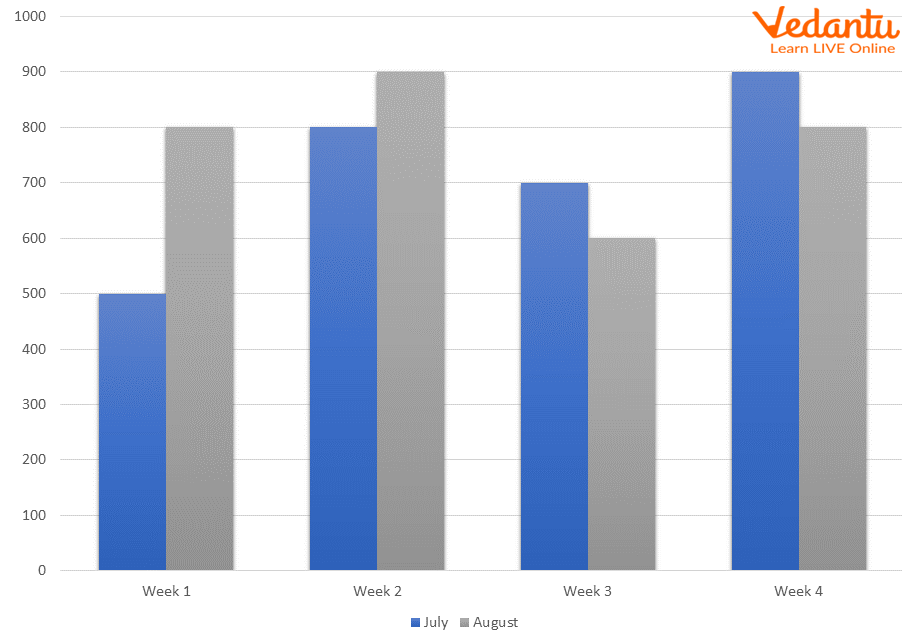Double Bar Graph

The height of each bar gives information, helping the children notice which columns have more objects and which columns have fewer objects. Bar graphs also help children sort and organize information.

## What is a Double Bar Graph?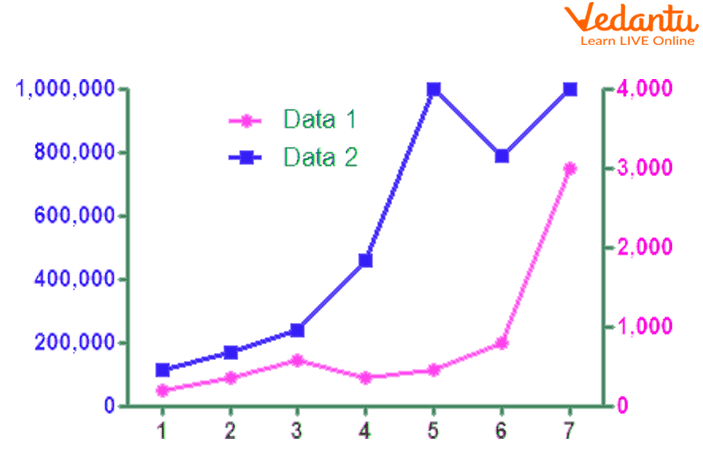Double Bar Graph

Bar graphs are a common visual representation. A bar graph is a graphical representation of data that uses lone bars of different heights.

A double bar graph is a visual representation of data that uses two parallel bars of varying heights. You can arrange the bars either vertically or horizontally. A double bar graph can be used to contrast two sets of data to visually compare and contrast between different sets of data using the double bar graph template.

## How to Make a Double Bar Graph?

To create a double bar graph, follow these steps:

• Make a data-filled table.

The Frankfurt annual high and low temperatures are shown in this table in degrees Celsius.

 Month Jan Feb Mar Apr May Jun Jul Aug Sep Oct Nov Dec Max 5 7 11 16 20 24 26 26 21 15 9 6 Min 0 0 2 6 10 13 15 15 11 7 3 1
• To see what kinds of numbers the y-axis should have, determine the range of the data.

The number 26 is the highest value while 0 is the lowest. This graph should be scaled such that 1 unit equals 10 degrees Celsius.

• Make a data plot.

Excel or other software programmes can be used to make a double bar graph. On a sheet of paper, it can also be completed manually.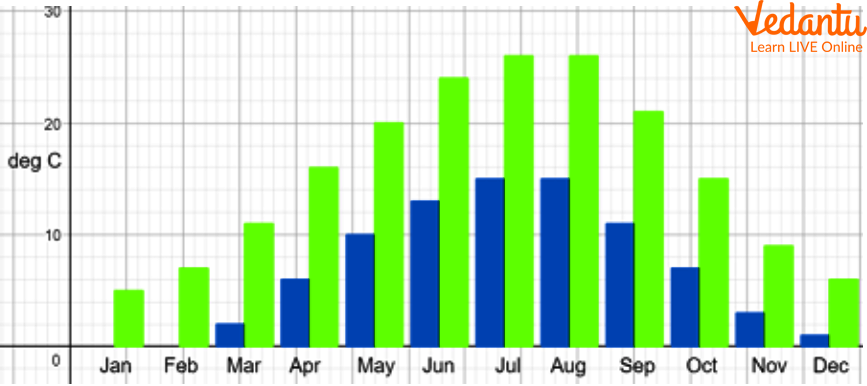Temperature- Month Bar Graph

• Give the x- and y-axes labels.

In this instance, the y-axis displays the temperature in degrees Celsius, and the x-axis displays the yearly months.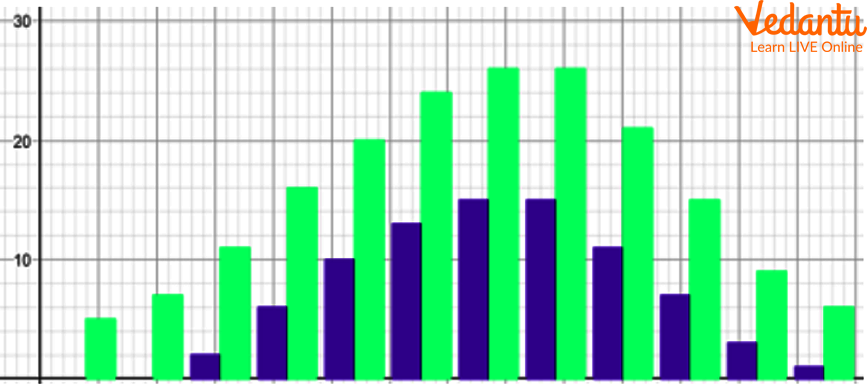Double Bar Graph

• Put a key.

The key here reveals that the highest temperatures are displayed in red and the lowest in green.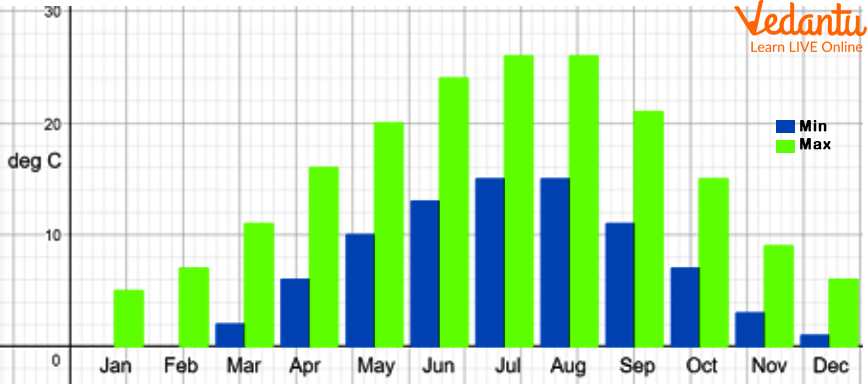A Double Bar Graph with a Key

The graph is described in the title.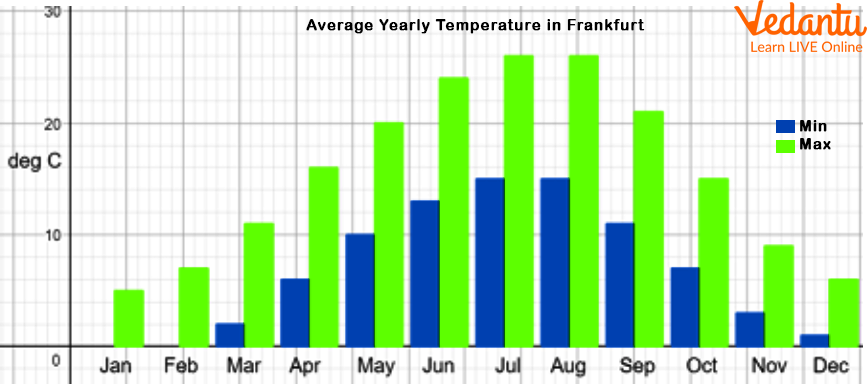A Double Bar Graph with Heading

## Double Bar Graph Example:Learning from Examples

Following are the double bar graph example-

Example 1.  What sport do girls like to play?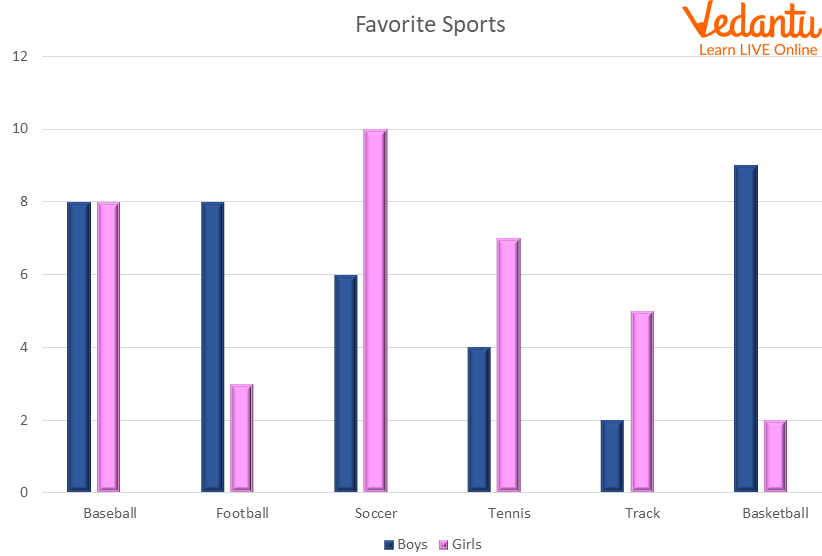Graph of Favorite Sports

Ans:  Look at the graph's pink-colored highest bar. The color of the bar indicates the girls' preferred sports.

Then confirm that the value on the y axis represents the greatest value for any female favorite sport. Soccer is the top choice in this instance with 10 females making that choice, which is also the highest number on the graph of girls' preferred sports.

Example 2.  Observe the given graph and answer the questions below.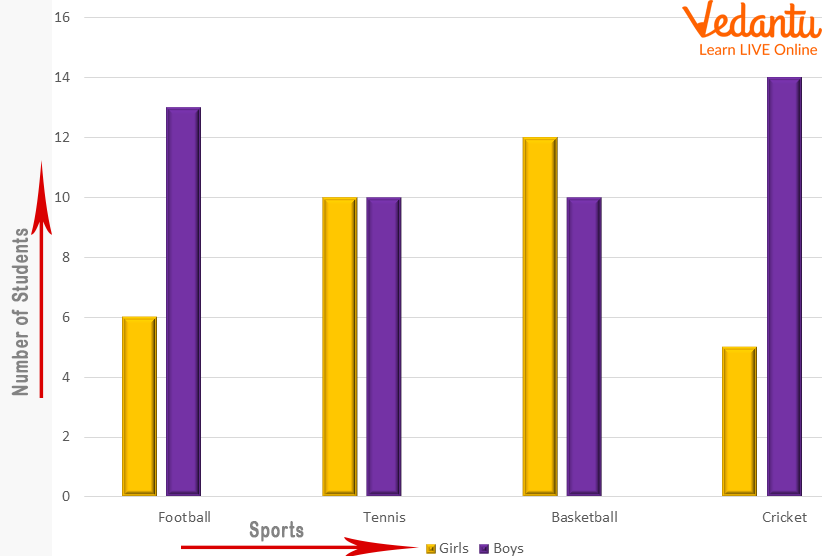Graph of Sports played by Girls and Boys

1. What sport do girls prefer to play the most?

2. Which sport do boys prefer the most?

Ans: Cricket

## Double Bar Graphs Compare Multiple Bar Graph: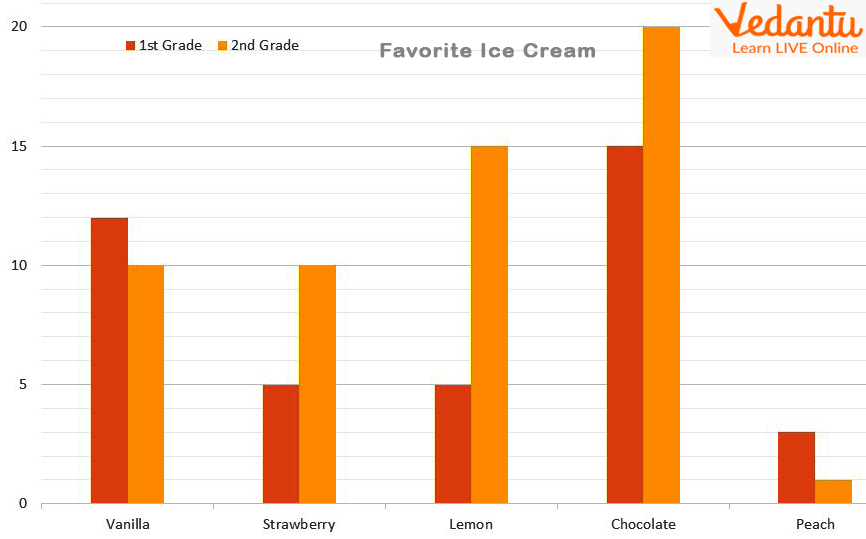Double Bar Graphs Compare Multiple

Let’s see double bar graphs compare multiple bar graph-

• Double Bar Graph - A double bar graph is used to display two sets of data on the same graph. We would utilize a double bar graph, for instance, to compare the number of hours that students worked in one month to another.

• Multiple Bar Graph - In a bar graph, there may occasionally be more than two sets of data to compare. A multiple bar graph can be utilized in that situation. Any number of data sets can be compared using a multiple bar graph.

## Summary

Two sets of data are shown on the same graph using a double bar graph. We would employ a double bar graph, for instance, to compare the number of hours that students worked in one month to another.

The double bar graph worksheets demonstrate how to read a double bar graph to find data and address issues.

Last updated date: 17th Sep 2023
Total views: 122.4k
Views today: 1.22k

## FAQs on Double Bar Graph

1. How to make a double bar graph and what is the title of a double bar graph?

The steps to follow when creating a double bar graph are:

• Create a table with data.

• Determine the range of the data.

• Plot the data.Label the x- and y-axis.

The double bar graph's title gives the viewer a broad picture of what is being measured and compared. A key will also be provided for a double bar graph. The trick to a double bar graph is to use two different colors to indicate the two groups that are being compared.

2. Simply put, what is a double bar graph and how is it read?

The graphical depiction of grouped data with two parameters provided for each category is called a double bar graph. Two bars are displayed for each category in a double bar graph. The two parameters for each group are represented by these two bars.

In order to read a double-bar graph, do the following:

• The subject(s) of the graph can be found by reading the title.

• To determine what the bars in the graph represent, look at the information in the key and on the side and bottom of the graph.

• To infer information about the graph's subjects, compare the lengths of the bars.